# AP Physics - Chapter 18 Powerpoint

Feb. 11, 2009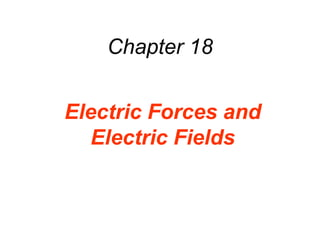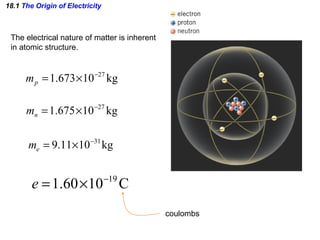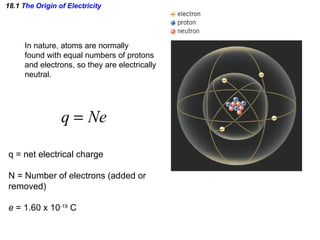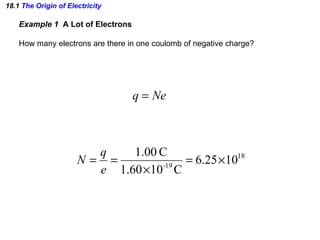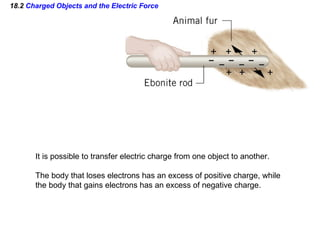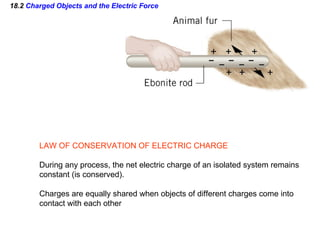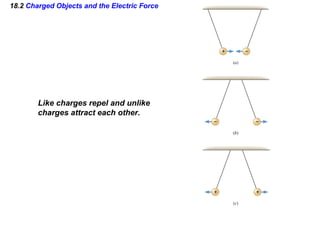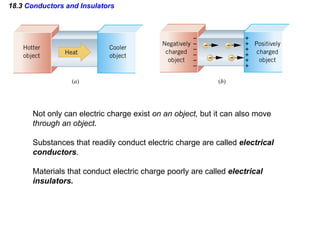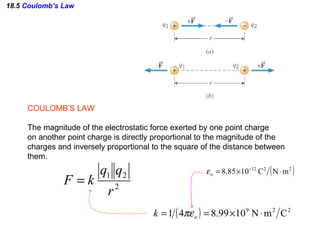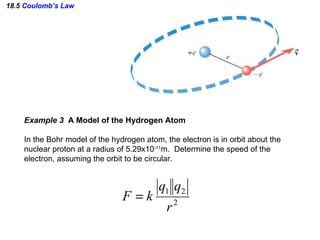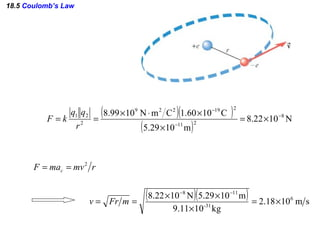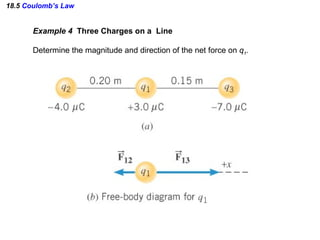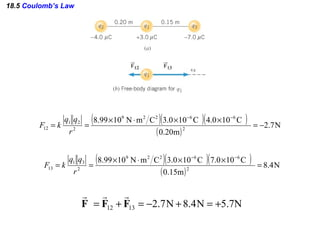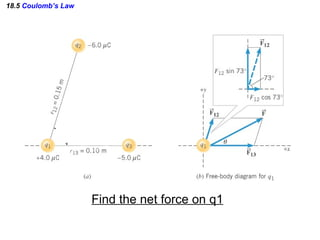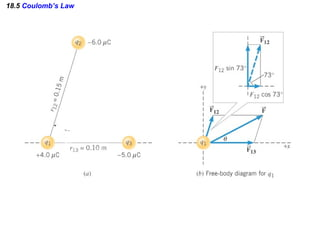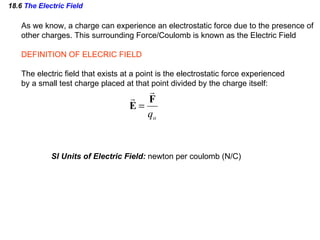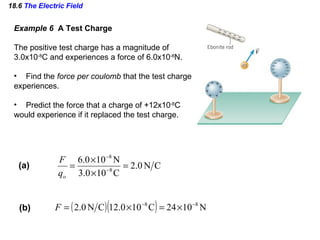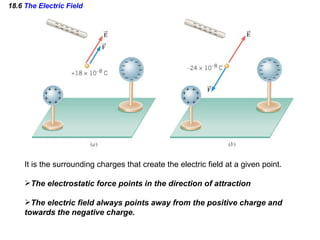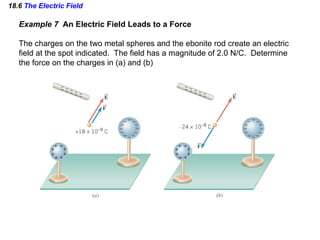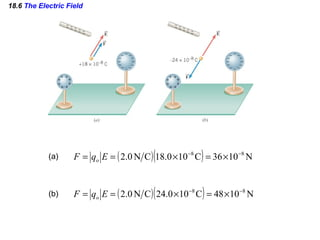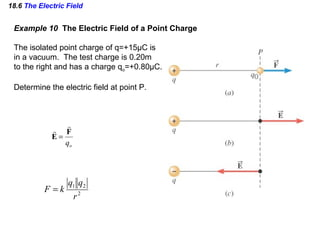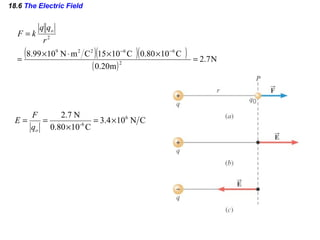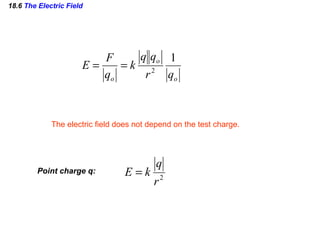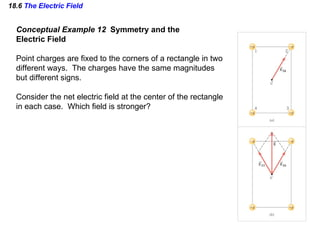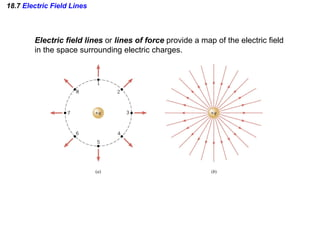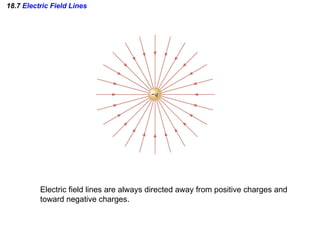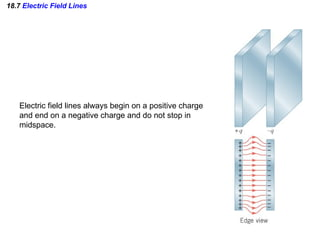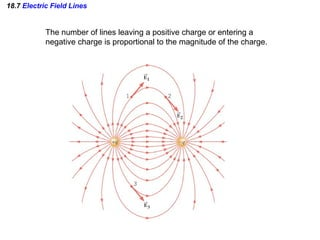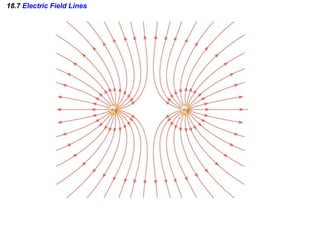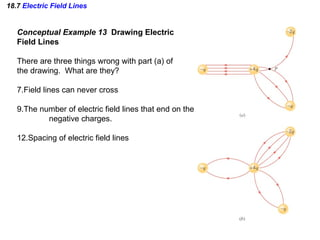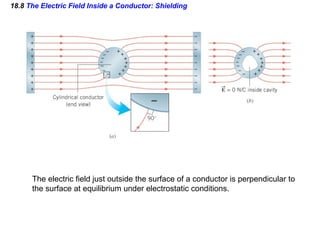1 of 31

### AP Physics - Chapter 18 Powerpoint

• 1. Chapter 18 Electric Forces and Electric Fields
• 2. 18.1 The Origin of Electricity The electrical nature of matter is inherent in atomic structure. coulombs
• 3. 18.1 The Origin of Electricity In nature, atoms are normally found with equal numbers of protons and electrons, so they are electrically neutral. q = net electrical charge N = Number of electrons (added or removed) e = 1.60 x 10 -19 C
• 4. 18.1 The Origin of Electricity Example 1 A Lot of Electrons How many electrons are there in one coulomb of negative charge?
• 5. 18.2 Charged Objects and the Electric Force It is possible to transfer electric charge from one object to another. The body that loses electrons has an excess of positive charge, while the body that gains electrons has an excess of negative charge.
• 6. 18.2 Charged Objects and the Electric Force LAW OF CONSERVATION OF ELECTRIC CHARGE During any process, the net electric charge of an isolated system remains constant (is conserved). Charges are equally shared when objects of different charges come into contact with each other
• 7. 18.2 Charged Objects and the Electric Force Like charges repel and unlike charges attract each other.
• 8. 18.3 Conductors and Insulators Not only can electric charge exist on an object, but it can also move through an object . Substances that readily conduct electric charge are called electrical conductors . Materials that conduct electric charge poorly are called electrical insulators.
• 9. 18.5 Coulomb’s Law COULOMB’S LAW The magnitude of the electrostatic force exerted by one point charge on another point charge is directly proportional to the magnitude of the charges and inversely proportional to the square of the distance between them.
• 10. 18.5 Coulomb’s Law Example 3 A Model of the Hydrogen Atom In the Bohr model of the hydrogen atom, the electron is in orbit about the nuclear proton at a radius of 5.29x10 -11 m. Determine the speed of the electron, assuming the orbit to be circular.
• 12. 18.5 Coulomb’s Law Example 4 Three Charges on a Line Determine the magnitude and direction of the net force on q 1 .
• 14. 18.5 Coulomb’s Law Find the net force on q1
• 16. 18.6 The Electric Field As we know, a charge can experience an electrostatic force due to the presence of other charges. This surrounding Force/Coulomb is known as the Electric Field DEFINITION OF ELECRIC FIELD The electric field that exists at a point is the electrostatic force experienced by a small test charge placed at that point divided by the charge itself: SI Units of Electric Field: newton per coulomb (N/C)
• 17.
• 18.
• 19. 18.6 The Electric Field Example 7 An Electric Field Leads to a Force The charges on the two metal spheres and the ebonite rod create an electric field at the spot indicated. The field has a magnitude of 2.0 N/C. Determine the force on the charges in (a) and (b)
• 20. 18.6 The Electric Field (a) (b)
• 21. 18.6 The Electric Field Example 10 The Electric Field of a Point Charge The isolated point charge of q=+15 μ C is in a vacuum. The test charge is 0.20m to the right and has a charge q o =+0.80 μ C. Determine the electric field at point P.
• 22. 18.6 The Electric Field
• 23. 18.6 The Electric Field The electric field does not depend on the test charge. Point charge q:
• 24. 18.6 The Electric Field Conceptual Example 12 Symmetry and the Electric Field Point charges are fixed to the corners of a rectangle in two different ways. The charges have the same magnitudes but different signs. Consider the net electric field at the center of the rectangle in each case. Which field is stronger?
• 25. 18.7 Electric Field Lines Electric field lines or lines of force provide a map of the electric field in the space surrounding electric charges.
• 26. 18.7 Electric Field Lines Electric field lines are always directed away from positive charges and toward negative charges.
• 27. 18.7 Electric Field Lines Electric field lines always begin on a positive charge and end on a negative charge and do not stop in midspace.
• 28. 18.7 Electric Field Lines The number of lines leaving a positive charge or entering a negative charge is proportional to the magnitude of the charge.
• 29. 18.7 Electric Field Lines
• 30.
• 31. 18.8 The Electric Field Inside a Conductor: Shielding The electric field just outside the surface of a conductor is perpendicular to the surface at equilibrium under electrostatic conditions.